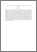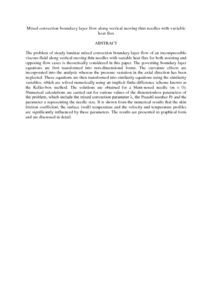# Mixed convection boundary layer flow along vertical moving thin needles with variable heat flux

## Citation

Ahmad, Syakila and Md Arifin, Norihan and Mohd Nazar, Roslinda and Pop, Ioan (2008) Mixed convection boundary layer flow along vertical moving thin needles with variable heat flux. Heat and Mass Transfer, 44 (4). pp. 473-479. ISSN 0947-7411

## Abstract

The problem of steady laminar mixed convection boundary layer flow of an incompressible viscous fluid along vertical moving thin needles with variable heat flux for both assisting and opposing flow cases is theoretically considered in this paper. The governing boundary layer equations are first transformed into non-dimensional forms. The curvature effects are incorporated into the analysis whereas the pressure variation in the axial direction has been neglected. These equations are then transformed into similarity equations using the similarity variables, which are solved numerically using an implicit finite-difference scheme known as the Keller-box method. The solutions are obtained for a blunt-nosed needle (m = 0). Numerical calculations are carried out for various values of the dimensionless parameters of the problem, which include the mixed convection parameter λ, the Prandtl number Pr and the parameter a representing the needle size. It is shown from the numerical results that the skin friction coefficient, the surface (wall) temperature and the velocity and temperature profiles are significantly influenced by these parameters. The results are presented in graphical form and are discussed in detail.Preview
PDF (Abstract)
Mixed convection boundary layer flow along vertical moving thin needles with variable heat flux.pdfView Item